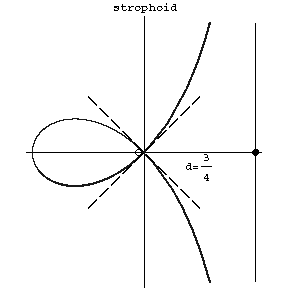# Strophoid

A third-order plane algebraic curve whose equation takes the form

$$y^2=x^2\frac{d+x}{d-x}$$

in Cartesian coordinates, and

$$\rho=-d\frac{\cos2\phi}{\cos\phi}$$

in polar coordinates. The coordinate origin is a node with tangents $y=\pm x$ (see Fig.). The asymptote is $x=d$. The area of the loop is

$$S=2d^2-\frac{1}{2\pi d^2}.$$

The area between the curve and the asymptote is

$$S_2=2d^2+\frac{1}{2\pi d^2}.$$

A strophoid is related to the so-called cusps (cf. Cusp).Figure: s090630a